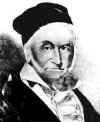#### You may also like### Purr-fection

What is the smallest perfect square that ends with the four digits 9009?### Old Nuts

In turn 4 people throw away three nuts from a pile and hide a quarter of the remainder finally leaving a multiple of 4 nuts. How many nuts were at the start?### Mod 7

Find the remainder when 3^{2001} is divided by 7.

# Modular Fractions

##### Age 16 to 18Challenge LevelIn arithmetic modulo 7 ($Z_7$) one integer is equal to another if the difference between the two integers is a multiple of 7. Rather like the days of the week, in $Z_7$ we only need seven numbers and they are usually named 0, 1, 2, 3, 4, 5 and 6.

If there is a solution in $Z_7$ to the equation $ax=1$ then we call this solution the inverse (or reciprocal) of $a$ and write it as $a^{-1}$ or ${1\over a}$. For example the fraction one half in arithmetic modulo 7 is the inverse of 2, that is the solution of $2x=1 \pmod 7$, namely the number 4 because $2\times 4 = 1 \pmod 7$.

What are the fractions one third, one quarter, one fifth and one sixth in arithmetic modulo 7?

Explain why all fractions in arithmetic modulo 7 are equivalent to one of the following set of numbers $\{0, 1, 2, 3, 4, 5, 6\}$.

Show that in $Z_7$ there are six different solutions to the equation

$${1\over x} + {1\over y} = {1\over {x+y}}.$$

Show that, by way of contrast, when working with real numbers this equation has no real solutions.